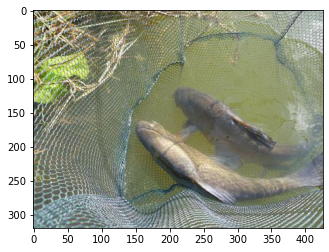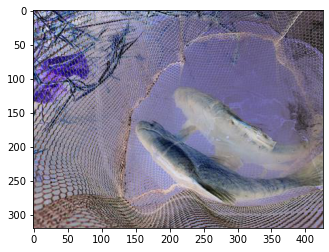In this tutorial we will first look at how we can use RandAugment to train our models using timm's training script. Next, we will also look at how one can call the rand_augment_transform function in timm and add RandAugment to custom training loops.

Finally, we will take a brief look at what RandAugment is and also look at the timms implementation of RandAugment in detail to understand the differences.

The research paper for RandAugment can be referred here.

## Training models with RandAugment using timm's training script

To train your models using randaugment, simply pass the --aa argument to the training script with a value. Something like:

python train.py ../imagenette2-320 --aa rand-m9-mstd0.5


Therefore, then by passing in the --aa argument with a value rand-m9-mstd0.5 means we will be using RandAugment where the magnitude of the augmentations operations is 9. Passing in a magnitude standard deviation means that the magnitute will vary based on the mstd value.

magnitude = random.gauss(magnitude, magnitude_std)


Thus this means that the magnitude varies as a gaussian distribution with standard deviation of mstd around the magnitude.

## Using RandAugment in custom training scripts

Don't want to use the training script from timm and just want to use the RandAugment method as an augmentation in your training script?

Just create a rand_augment_transform as shown below but make sure that your dataset applies this transform to the input when the input image is a PIL.Image and not torch.tensor. That is, this method only works on PIL.Images and not tensors.

The normalization and conversion to tensor operation can be performed after the RandAugment augmentation has been applied.

Let's see a quick example of the rand_augment_transform function in timm in action!

from timm.data.auto_augment import rand_augment_transform
from PIL import Image
from matplotlib import pyplot as plt

tfm = rand_augment_transform(
config_str='rand-m9-mstd0.5',
hparams={'translate_const': 117, 'img_mean': (124, 116, 104)}
)

x   = Image.open("../../imagenette2-320/train/n01440764/ILSVRC2012_val_00000293.JPEG")
plt.imshow(x)


Let's visualize the original image x.

plt.imshow(x)

<matplotlib.image.AxesImage at 0x7f8f2d7a2520>Great! It's an image of a "tench". (If you're not aware about what a "tench" is, you're not a true deep learning practitioner)

Let's now visualize the transformed version of the image.

plt.imshow(tfm(x))

<matplotlib.image.AxesImage at 0x7f8f2809f430>As we can see, the rand_augment_transform above is performing data augmentation on our input image x.

## What is RandAugment?

In this section we will first look into what RandAugment is and later in section 1.2 we will look into the timms implementation of RandAugment. Feel free to skip as it does not really add any more information but only explains how timm implements RandAugment.

From the paper, RandAugment can be implemented in numpy like so:

transforms = [
’Identity’, ’AutoContrast’, ’Equalize’,
’Rotate’, ’Solarize’, ’Color’, ’Posterize’,
’Contrast’, ’Brightness’, ’Sharpness’,
’ShearX’, ’ShearY’, ’TranslateX’, ’TranslateY’]

def randaugment(N, M):
"""Generate a set of distortions.
Args:
N: Number of augmentation transformations to
apply sequentially.
M: Magnitude for all the transformations.
"""
sampled_ops = np.random.choice(transforms, N)
return [(op, M) for op in sampled_ops]


Basically we have a list of transforms, and from that list we select N transforms. Next, we apply that operation with a magnitude of M to the input image. And that's really it. That's RandAugment. Let's have a look at how timm implements this.

## timms implementation of RandAugment

### rand_augment_transform

In this section we will be taking a deep dive inside the rand_augment_transform function. Let's take a look at the source code:

def rand_augment_transform(config_str, hparams):
"""
Create a RandAugment transform

:param config_str: String defining configuration of random augmentation. Consists of multiple sections separated by
dashes ('-'). The first section defines the specific variant of rand augment (currently only 'rand'). The remaining
sections, not order sepecific determine
'm' - integer magnitude of rand augment
'n' - integer num layers (number of transform ops selected per image)
'w' - integer probabiliy weight index (index of a set of weights to influence choice of op)
'mstd' -  float std deviation of magnitude noise applied
'inc' - integer (bool), use augmentations that increase in severity with magnitude (default: 0)
Ex 'rand-m9-n3-mstd0.5' results in RandAugment with magnitude 9, num_layers 3, magnitude_std 0.5
'rand-mstd1-w0' results in magnitude_std 1.0, weights 0, default magnitude of 10 and num_layers 2

:param hparams: Other hparams (kwargs) for the RandAugmentation scheme

:return: A PyTorch compatible Transform
"""
magnitude = _MAX_LEVEL  # default to _MAX_LEVEL for magnitude (currently 10)
num_layers = 2  # default to 2 ops per image
weight_idx = None  # default to no probability weights for op choice
transforms = _RAND_TRANSFORMS
config = config_str.split('-')
assert config == 'rand'
config = config[1:]
for c in config:
cs = re.split(r'(\d.*)', c)
if len(cs) < 2:
continue
key, val = cs[:2]
if key == 'mstd':
# noise param injected via hparams for now
hparams.setdefault('magnitude_std', float(val))
elif key == 'inc':
if bool(val):
transforms = _RAND_INCREASING_TRANSFORMS
elif key == 'm':
magnitude = int(val)
elif key == 'n':
num_layers = int(val)
elif key == 'w':
weight_idx = int(val)
else:
assert False, 'Unknown RandAugment config section'
ra_ops = rand_augment_ops(magnitude=magnitude, hparams=hparams, transforms=transforms)
choice_weights = None if weight_idx is None else _select_rand_weights(weight_idx)
return RandAugment(ra_ops, num_layers, choice_weights=choice_weights)


The basic idea behind the function above is this - "Based on the config str passed, update the hparams parameter and also set the value of the variable magnitude if passed, unless it remains the default value _MAX_LEVEL which is 10.0.

Also set the transforms variable to _RAND_TRANSFORMS. _RAND_TRANSFORMS is a list of transforms to choose from similar to the paper that looks like

_RAND_TRANSFORMS = [
'AutoContrast',
'Equalize',
'Invert',
'Rotate',
'Posterize',
'Solarize',
'Color',
'Contrast',
'Brightness',
'Sharpness',
'ShearX',
'ShearY',
'TranslateXRel',
'TranslateYRel',
#'Cutout'  # NOTE I've implement this as random erasing separately
]


Once the hparams, magnitude and transforms variables have been set, next, call the rand_augment_ops function to set a value for the variable ra_ops. Finally we call return an instance RandAugment class based on these variables.

So let's next look into rand_augment_ops function and RandAugment class.

### rand_augment_ops

The complete source code of this function looks something like:

def rand_augment_ops(magnitude=10, hparams=None, transforms=None):
hparams = hparams or _HPARAMS_DEFAULT
transforms = transforms or _RAND_TRANSFORMS
return [AugmentOp(
name, prob=0.5, magnitude=magnitude, hparams=hparams) for name in transforms]


Basically, it creates an instance of the AugmentOp class. So, all the fun is inside the AugmentOp class. Let's take a look at it.

### AugmentOp

Let's take a look at the source code of this class.

class AugmentOp:

def __init__(self, name, prob=0.5, magnitude=10, hparams=None):
hparams = hparams or _HPARAMS_DEFAULT
self.aug_fn = NAME_TO_OP[name]
self.level_fn = LEVEL_TO_ARG[name]
self.prob = prob
self.magnitude = magnitude
self.hparams = hparams.copy()
self.kwargs = dict(
fillcolor=hparams['img_mean'] if 'img_mean' in hparams else _FILL,
resample=hparams['interpolation'] if 'interpolation' in hparams else _RANDOM_INTERPOLATION,
)

# If magnitude_std is > 0, we introduce some randomness
# in the usually fixed policy and sample magnitude from a normal distribution
# with mean magnitude and std-dev of magnitude_std.
# NOTE This is my own hack, being tested, not in papers or reference impls.
self.magnitude_std = self.hparams.get('magnitude_std', 0)

def __call__(self, img):
if self.prob < 1.0 and random.random() > self.prob:
return img
magnitude = self.magnitude
if self.magnitude_std and self.magnitude_std > 0:
magnitude = random.gauss(magnitude, self.magnitude_std)
magnitude = min(_MAX_LEVEL, max(0, magnitude))  # clip to valid range
level_args = self.level_fn(magnitude, self.hparams) if self.level_fn is not None else tuple()
return self.aug_fn(img, *level_args, **self.kwargs)


Above, we already know that the value of self.prob is 0.5. Therefore, calling this class will return the img 50% of the time and actually perform the actual self.aug_fn 50% of the time.

You might ask what is this self.aug_fn? Remember that the transforms was a list of _RAND_TRANFORMS as below:

_RAND_TRANSFORMS = [
'AutoContrast',
'Equalize',
'Invert',
'Rotate',
'Posterize',
'Solarize',
'Color',
'Contrast',
'Brightness',
'Sharpness',
'ShearX',
'ShearY',
'TranslateXRel',
'TranslateYRel',
#'Cutout'  # NOTE I've implement this as random erasing separately
]


And that we create a list of instances of AugmentOp like so [AugmentOp(name, prob=0.5, magnitude=magnitude, hparams=hparams) for name in transforms] for each of the transforms that get's returned by rand_augment_ops.

Well, the self.aug_fn actually first uses the NAME_TO_OP dictionary to convert the name to operation.

This NAME_TO_OP is nothing but a dictionary that links each of the _RAND_TRANSFORMS names to their respective function implementations inside timm.

NAME_TO_OP = {
'AutoContrast': auto_contrast,
'Equalize': equalize,
'Invert': invert,
'Rotate': rotate,
'Posterize': posterize,
'PosterizeIncreasing': posterize,
'PosterizeOriginal': posterize,
'Solarize': solarize,
'SolarizeIncreasing': solarize,
'Color': color,
'ColorIncreasing': color,
'Contrast': contrast,
'ContrastIncreasing': contrast,
'Brightness': brightness,
'BrightnessIncreasing': brightness,
'Sharpness': sharpness,
'SharpnessIncreasing': sharpness,
'ShearX': shear_x,
'ShearY': shear_y,
'TranslateX': translate_x_abs,
'TranslateY': translate_y_abs,
'TranslateXRel': translate_x_rel,
'TranslateYRel': translate_y_rel,
}


So in summary, this AugmentOp is nothing but a wrapper on top of thie self.aug_fn that accepts an img and only performs the self.aug_fn on the img 50% of the times. Otherwise, it just returns the img unchanged.

Great so this ra_ops variable inside the rand_augment_transform function is nothing but a list of instances of the AugmentOp class that just means that we apply the given augmentation function 50% of the time to the image.

Finally, as we saw in the source code of rand_augment_transform, what get's returned is actually an instance of RandAugment class that accepts the ra_ops, choice_weights and num_layers as arguments. So let's took at it next.

### RandAugment

The complete source code of this class looks like:

class RandAugment:
def __init__(self, ops, num_layers=2, choice_weights=None):
self.ops = ops
self.num_layers = num_layers
self.choice_weights = choice_weights

def __call__(self, img):
# no replacement when using weighted choice
ops = np.random.choice(
self.ops, self.num_layers, replace=self.choice_weights is None, p=self.choice_weights)
for op in ops:
img = op(img)
return img


As already mentioned before, the ra_ops that get's passed to RandAugment is nothing but a list of instances of AugmentOp wrapper around the various transforms in _RAND_TRANSFORMS, so this ops looks something like:

ops = [<timm.data.auto_augment.AugmentOp object at 0x7f7a03466990>, <timm.data.auto_augment.AugmentOp object at 0x7f7a03466c50>, <timm.data.auto_augment.AugmentOp object at 0x7f7a03466650>, <timm.data.auto_augment.AugmentOp object at 0x7f7a034666d0>, <timm.data.auto_augment.AugmentOp object at 0x7f7a03466e10>, <timm.data.auto_augment.AugmentOp object at 0x7f7a03466490>, <timm.data.auto_augment.AugmentOp object at 0x7f7a03466750>, <timm.data.auto_augment.AugmentOp object at 0x7f7a034667d0>, <timm.data.auto_augment.AugmentOp object at 0x7f7a03466410>, <timm.data.auto_augment.AugmentOp object at 0x7f7a03466710>, <timm.data.auto_augment.AugmentOp object at 0x7f7a03466190>, <timm.data.auto_augment.AugmentOp object at 0x7f7a03466450>, <timm.data.auto_augment.AugmentOp object at 0x7f7a034664d0>, <timm.data.auto_augment.AugmentOp object at 0x7f7a03466150>, <timm.data.auto_augment.AugmentOp object at 0x7f7a034661d0>]


As can be seen, the ops is nothing a but a list of AugmentOp instances. Basically, each transform is wrapped around by this AugmentOp class which means that the transform only get's applied 50% of the time.

Next, for each img, we select num_layers random augmentation and apply it to the image as in the __call__ method of this class.

ops = np.random.choice(
self.ops, self.num_layers, replace=self.choice_weights is None, p=self.choice_weights)
for op in ops:
img = op(img)


Finally, we return this augmented image.